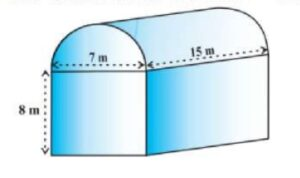Q) Shanta runs an industry in a shed which was in the shape of a cuboid surmounted by half cylinder. The dimensions of the base were 15 m x 7m x 8 m. The diameter of the half cylinder was 7 m and length was 15 m.(i) Find the volume of the air that the shed can hold.
(ii) If the industry requires machinery which would occupy a total space of 300 m³ and there are 20 workers each of whom would occupy 0.08 m³ space on an average, how much air would be in the shed when it is working?
(iii) Find the surface area of the cuboidal part.
OR
Find the surface area of the cylindrical part.

Ans:

We are given that, Cuboid size (l, b, h) = 15 m  x 7 m x 8 m

Cylinder’s diameter = 7m and height = 15 m

(i) Volume of Air:

Since, Volume of the air will be equal to volume of the shed.

and volume of shed = Volume of cuboid + Volume of half cylinder

Volume of cuboid = l x b x h = 15 x 7 x 8 = 840 m3

Volume of half cylinder =r2 h) =x 15

== 288.75 m3

Hence, Volume of shed = 840 + 288.75 = 1128.75 m3

Therefore, the shed will have 1,128.75 mair.

(ii) Volume of Air in the shed with machinery & workers inside:

When machinery and workers are inside the shed, that much volume will get consumed by them and air will be there only in remaining space.

Hence, let’s calculate the volume of space taken up:

Volume of space consumed by machine: 300 m3

Volume of space consumed by workers: 20 x 0.08 = 1.6 m3

Total space consumed = space consumed by machine + space consumed by workers

= 300 + 1.6 = 301.6 m3

Hence, the remaining volume in the shed = total space of the shed – space consumed by machine & workers = 1128.75 – 301.6 =  827.15 m3

Therefore there will 827.15 mof air when the machine will be working.

(iii) Surface area of the cuboidal part:

The surface area of the cuboidal part will be = surface area of 4 walls

(roof surface area is dropped because the shed’s roof is half cylindrical, not the roof of cuboid; floor is not counted in the shed)

Therefore, total surface area of cuboidal part = 2 h (l + b)

= 2 x 8 (15 + 7) = 354 m3

Therefore, total surface area of cuboidal part is 354 m3

OR
(iii) Surface area of the cylindrical part:

We know that the surface area of a cylinder = 2 π r (h + r)

Since the cylindrical part in the shape is half cylinder, we need to calculate the total surface area of the half cylinder. i.e.[2 π r (h + r)] =  π r (h + r)

== 11 (15 + 3.5) = 203.5 m2

Therefore, the surface area of the cylindrical part is 203.5 m2.

Scroll to Top